# Johannes Baeumler: Chemical distances for long-range percolation

Abstract:

Consider long-range percolation on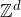, where there is an edge between two points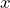and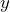with probability asymptotic to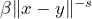, independent of all other edges, for some positive parameters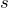and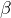. In this talk, we will focus on the metric properties of the long-range percolation graph. The chemical distance between two pointsandis the number of steps one needs to make in order to go fromto. For different values of, there are different regimes of how the chemical distance scales with the Euclidean distance. The transitions between these regimes happen at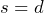and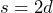. After an overview of previous work, we will focus on the case. We will show that for, for each dimension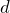and for each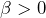, there exists a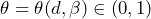such that the chemical distance betweenandis of order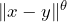. We will also discuss how the exponentdepends on the parameter.

______________________

Based on

https://arxiv.org/abs/2208.04800

https://arxiv.org/abs/2208.04793

## Video